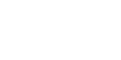When you run a complex chain need to prepare a sketch with a sketch of the motif. Sketches of chains need to perform on the graph paper: this will greatly simplify the selection of size of straw ribbons and pegging.
Complex chains differ from the simple fact that they can have multiple levels and consist of several types of elements. The rhythm of the sequence elements of a simple, often using one (figure 4.26). Elements, as can be seen from the figure, belong to the first group of geometric elements. They are located in 2-3 tiers, which allows to significantly expand the variety of chains, even made on the basis of one geometric element (figure 4.26 a, b, C). The combination of several elements expands the possible combinations many times (figure 4.26 d, e, f, g, h).
Consider the order of execution of multiple complex chains of the examples given in figure 4.26.A complex chain on the basis of a rectangle (figure 4.27).
Materials:
5-7 thatched strips of width 6 mm (figure 4.27 a), length 15— 18 cm
Execution of the work.
1. On the prepared surface with a pencil draw a vertical axis and line marking (figure 4.27 b). The line will be placed in two layers, using the same intervals. For the samples, the length of lines and distance between them need to choose similar to the examples of the simple chaining: line length — 15 cm, the distance between the lines corresponds to the width of the straw strips (6 mm).
2. Start to lay out the chain axis (figure 4.27). The length of rectangular elements can be selected arbitrarily. When placing elements, the surface of oil varnish and grind each element of the handle of the knife. In the example, the elements rhythmically move from one level to another, following the pattern (figure 4.27 d, e). Type chain for all length lines.
A complex chain on the basis of a square (figure 4.28). The following example considers a complex chain composed of squares. In the chain uses a single element — the "square" directly and "on the edge". This arrangement creates the illusion of multiple items.The chain is three-tiered and has a more complex rhythm of alternation of elements than in the previous example. Therefore, the order of operations will be slightly different from the previous example.
Materials:
5-7 thatched strips of width 6 mm (figure 4.28 a), length 15— 18 cm
Execution of the work.
1. Carry out the marking of the surface. Draw a vertical axis, perpendicular to the two parallel lines, which determines the position of squares; the boundary lines will be from them at a distance equal to the diagonal of the square (figure 4.28 b).
A set of chains start with uploading the middle-tier from the vertical axis to the right and left, alternating with his / her direct squares with the set "on the edge" (figure 4.28).
3. Every straight square top and bottom complement the square on an edge, forming the side tiers of the chain (figure 4.28 g).
A complex chain on the basis of a rectangle and a square (figure 4.29). This chain consists of two elements — a square and a rectangle, both of which have equal width (figure 4.29 a).Materials:
5-7 thatched strips of width 6 mm (figure 4.29 a), length 15— 18 cm
Execution of the work.
1. Follow the layout lines on the plane. Chain located in three tiers. The distance between the lines is identical and equal to the width of the main elements (figure 4.29 b).
2. Set of chain start from rectangular elements, which are located in the extreme tiers (figure 4.29).
3. Following diagram, lay out squares in middle layer (figure 4.29 d), then side (figure 4.29 d), again on average (figure 4.29 e).
4. Repeat the sequence of putting some elements according to figure until the end of the marking lines.
A complex chain on the basis of a rhombus and an elongated rectangle (figure 4.30). Despite the fact that the chain is located along the zigzag line, a conditional, we assume its three-tiered.Materials:
2-3 tape width of 6 mm and 3-4 strips 2 mm in width. the Length of all belts 15 to 18 cm (figure 4.30 a).
Execution of the work.
1. Draw the vertical axis and the line marking. Define the following distance between the lines: the average tier — 5 mm, lateral tiers — 7 mm. On the lines of middle-tier zig-zag mark the points that will determine the position of the rectangles. The distance between two adjacent points on a straight line approximately 25 mm, for direct neighboring points are located at the same distance with a shift equal to half the length (13 mm) (figure 4.30 b). These dimensions are easier to reconcile on graph paper, draw an arrangement of lines, features, and measure the distance between them.
2. Set of chain should start with putting some zigzag of elongated rectangles to cue points (figure 4.30 b); keep diagonal corners coincide with the markup as shown in the figure. Straw tape apply as shown in the diagram, and cut off at a right angle at the point. Zigzag finish lines.
3. Of the ribbons of the second size cut of the diamonds, which must be placed in the valleys of the zigzag with a gap of 1.5 mm (figure 4.30 d). Small diagonal diamonds coincides with the perpendicular.